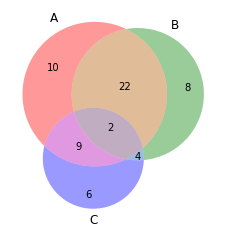You can draw a basic venn diagram of 3 groups using the `venn3()` function of matplotlib. `subsets` parameter takes the values of each groups and their intersections.

``````# Import the library
import matplotlib.pyplot as plt
from matplotlib_venn import venn3

# Make the diagram
venn3(subsets = (10, 8, 22, 6,9,4,2))
plt.show()``````## Contact & Edit

👋 This document is a work by Yan Holtz. You can contribute on github, send me a feedback on twitter or subscribe to the newsletter to know when new examples are published! 🔥